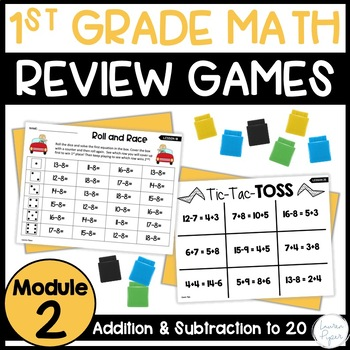First Grade Eureka Math Engage NY: Module 2 Games and Activities1st, Homeschool
Subjects
Standards
Resource Type
Formats Included
• PDF
Pages
137 pages

Also included in

1. This is the perfect supplemental product for your First Grade Eureka Math Curriculum! Included in this bundle are games and activities for EVERY SINGLE LESSON in the 1st Grade Eureka Curriculum. The activities can be used in whole group, small groups, or centers. They are easy prep and most activi
\$69.00
\$77.00
Save \$8.00
2. All you need for teaching First Grade Eureka Math Module 2 is in this BUNDLE! These supplemental resources for Eureka Math/Engage NY are student-friendly and align perfectly with the first grade curriculum. Included in this bundle: -First Grade Eureka Math Module 2 Games and Activities: A game for e
\$23.00
\$27.00
Save \$4.00

Description

Are you looking for supplements for your First Grade Eureka Math curriculum? These First Grade Eureka Math Module 2 Games provide FUN practice to help your students master the module 2 concepts. It includes a game, activity, or craft for EVERY lesson in Grade 1 Module 2 of Eureka Math/Engage NY.

These activities are perfect to use for small group, whole group, or centers! They are easy prep and most games require little to no materials. Your students will be engaged and excited to learn the strategies taught in First Grade Eureka Math Module 2!

You may purchase my other modules OR save big with the BUNDLE listed above!

Module 1

Module 3

Module 4

Module 5

Module 6

Do you need homework, review, or topic quizzes for your Eureka curriculum? Check out my First Grade Eureka Math Review and Assessments and be set for the whole year!

Total Pages
137 pages
N/A
Teaching Duration
N/A
Report this Resource to TpT
Reported resources will be reviewed by our team. Report this resource to let us know if this resource violates TpT’s content guidelines.

Standards

to see state-specific standards (only available in the US).
Add and subtract within 20, demonstrating fluency for addition and subtraction within 10. Use strategies such as counting on; making ten (e.g., 8 + 6 = 8 + 2 + 4 = 10 + 4 = 14); decomposing a number leading to a ten (e.g., 13 - 4 = 13 - 3 - 1 = 10 - 1 = 9); using the relationship between addition and subtraction (e.g., knowing that 8 + 4 = 12, one knows 12 - 8 = 4); and creating equivalent but easier or known sums (e.g., adding 6 + 7 by creating the known equivalent 6 + 6 + 1 = 12 + 1 = 13).
Understand subtraction as an unknown-addend problem. For example, subtract 10 – 8 by finding the number that makes 10 when added to 8.
Apply properties of operations as strategies to add and subtract. If 8 + 3 = 11 is known, then 3 + 8 = 11 is also known. (Commutative property of addition.) To add 2 + 6 + 4, the second two numbers can be added to make a ten, so 2 + 6 + 4 = 2 + 10 = 12. (Associative property of addition.)
Solve word problems that call for addition of three whole numbers whose sum is less than or equal to 20, e.g., by using objects, drawings, and equations with a symbol for the unknown number to represent the problem.
Use addition and subtraction within 20 to solve word problems involving situations of adding to, taking from, putting together, taking apart, and comparing, with unknowns in all positions, e.g., by using objects, drawings, and equations with a symbol for the unknown number to represent the problem.